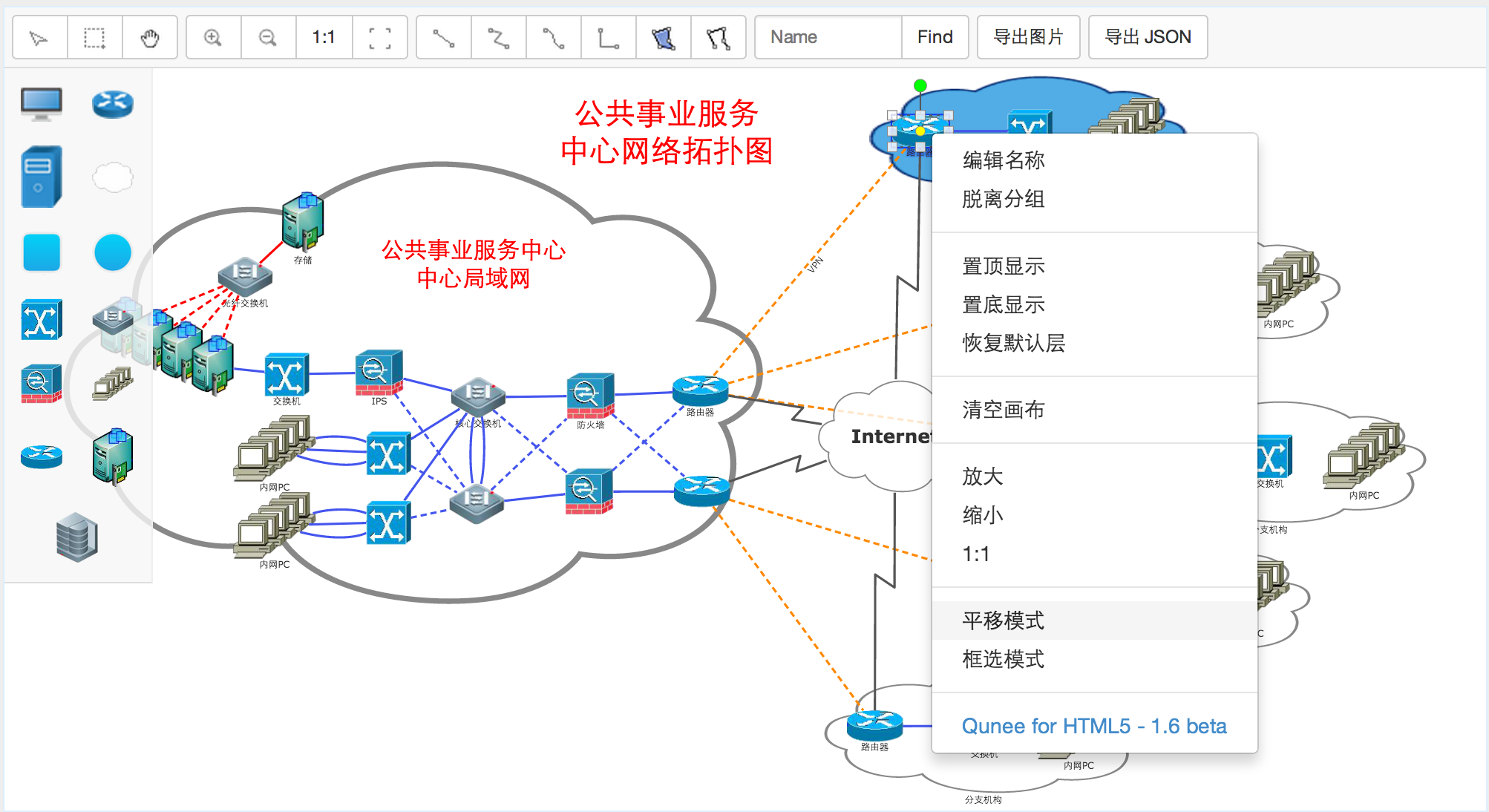# 拓扑图编辑器（2）

2014-09-01

## 新增多种交互模式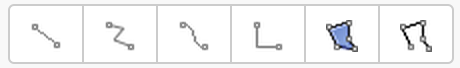### 创建连线交互

``````Q.Consts.INTERACTION_MODE_CREATE_SIMPLE_EDGE = "create.simple.edge";
Q.Consts.INTERACTION_MODE_CREATE_EDGE = "create.edge";
Q.Consts.INTERACTION_MODE_CREATE_SHAPE = "create.shape";
Q.Consts.INTERACTION_MODE_CREATE_LINE = "create.line";
``````

``````graph.interactionMode = Q.Consts.INTERACTION_MODE_CREATE_EDGE;
``````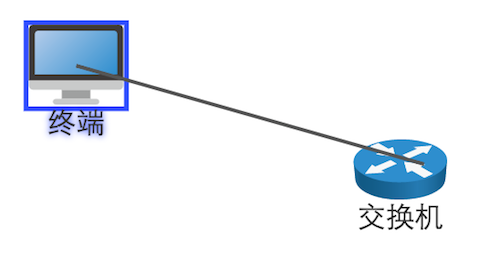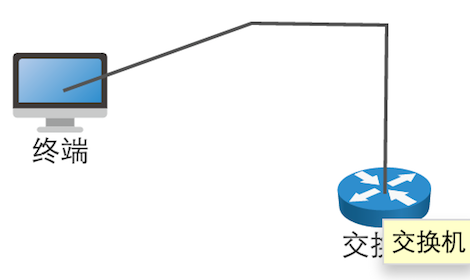### 连线编辑

``````graph.editable = true;
graph.interactionMode = Q.Consts.INTERACTION_MODE_DEFAULT;
``````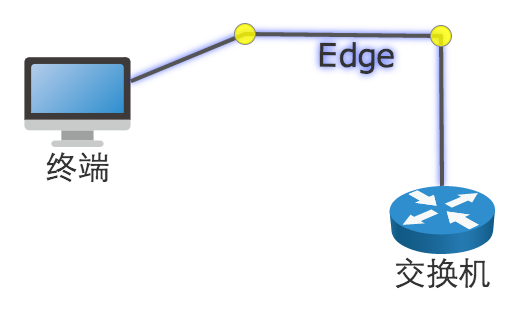### 创建多边形

``````graph.interactionMode = Q.Consts.INTERACTION_MODE_CREATE_SHAPE;
``````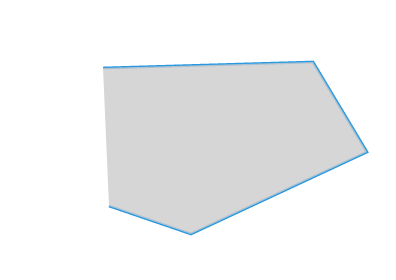### 创建多线段

``````graph.interactionMode = Q.Consts.INTERACTION_MODE_CREATE_LINE;
``````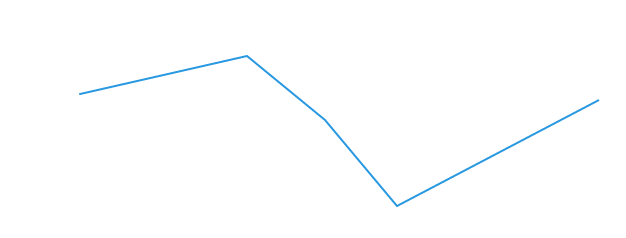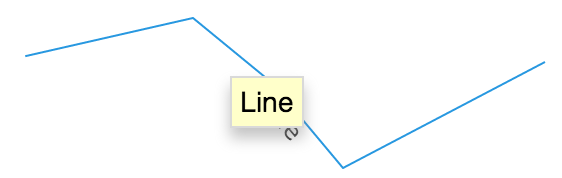### 编辑多边形

``````graph.editable = true;
graph.interactionMode = Q.Consts.INTERACTION_MODE_DEFAULT;
``````

## 右键菜单交互

``````graph.html.oncontextmenu = function(evt){
Q.stopEvent(evt);
}
onstart: function (evt) {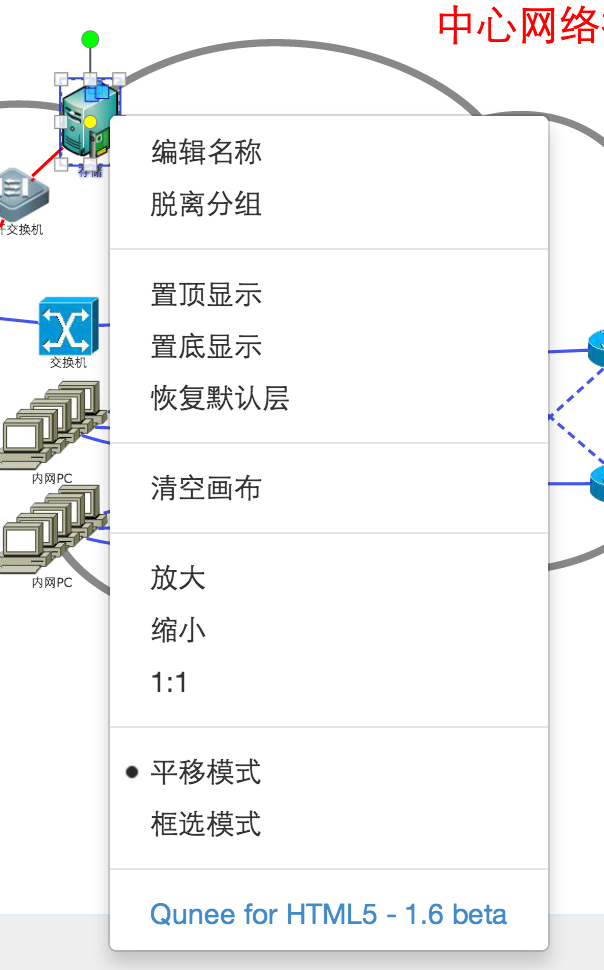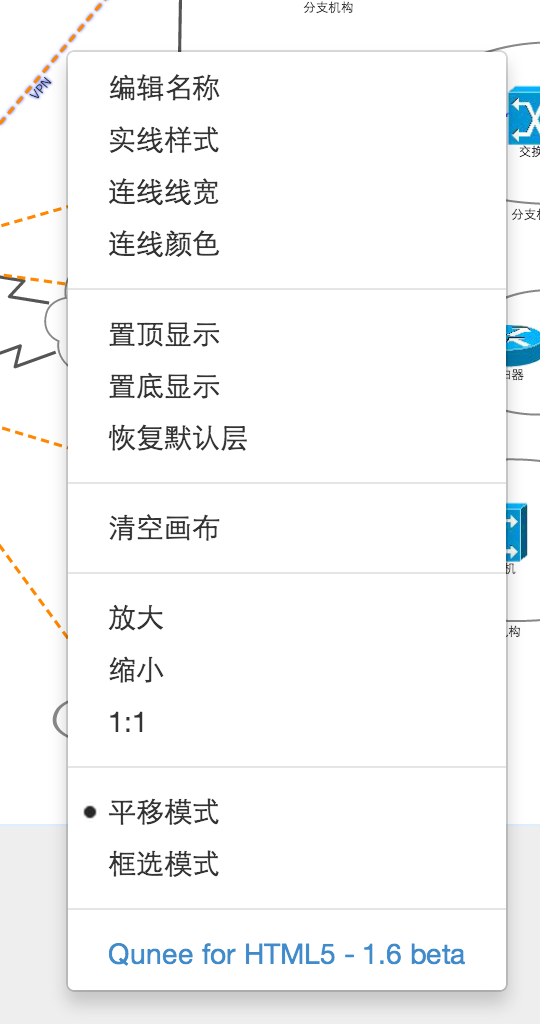http://demo.qunee.com/editor/Editor1.6.html